On-line GuidesAll GuideseBook StoreiOS / AndroidLinux for BeginnersOffice ProductivityLinux InstallationLinux SecurityLinux UtilitiesLinux VirtualizationLinux KernelSystem/Network AdminProgrammingScripting LanguagesDevelopment ToolsWeb DevelopmentGUI Toolkits/DesktopDatabasesMail SystemsopenSolarisEclipse DocumentationTechotopia.comVirtuatopia.comHow To GuidesVirtualizationGeneral System AdminLinux SecurityLinux FilesystemsWeb ServersGraphics & DesktopPC HardwareWindowsProblem SolutionsPrivacy PolicyGrokking The Gimp
Previous Page Home Next Page

## 5.6.2 The Addition, Subtract, and Difference Blending Modes

Addition, Subtract, and Difference are blending modes that add and subtract foreground and background pixel values in RGB colorspace. Figure  5.14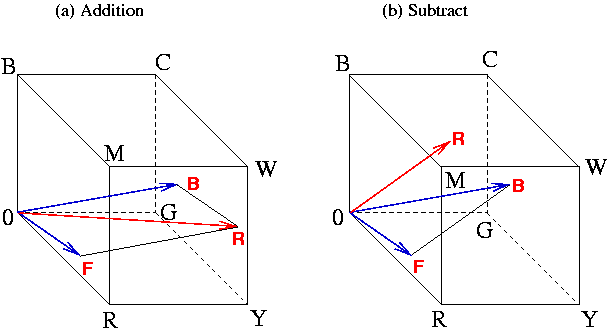illustrates the effect of addition and subtraction for two pixels in the RGB cube.

The Addition blending mode works as follows. Given a foreground and background pixel, represented by the RGB vectors F=[r1,g1,b1]and B=[r2,g2,b2], the pixel obtained from the Addition blending mode is R=F+B=[r1+r2,g1+g2,b1+b2]. Thus, in Figure  5.14(a), the two blue arrows represent a foreground and background pixel, and the red arrow is the vector sum of the two. Addition always produces a resultant color that is as light or lighter than either the foreground or background colors. This is because the vector sum must have a projection onto the neutral axis that is closer to white than the projection for either the background or foreground colors.

In the event that the vector sum produces a result outside of the color cube (that is, by producing any RGB component greater than 255), its value is clipped to the surface of the cube. The equation describing the Addition blending mode is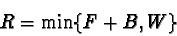where W is the vector [255,255,255] and the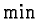function performs component-wise minimization of the two vectors.

Cyan, magenta, and yellow are the sums of green and blue, red and blue, and red and green, respectively. Thus, cyan, magenta, and yellow appear lighter than red, green, or blue because they project higher up onto the neutral axis. Furthermore, any of these secondary colors (cyan, magenta, yellow) summed with the complementary primary color (red, green, blue) produces white, the lightest color of all.

Figure  5.15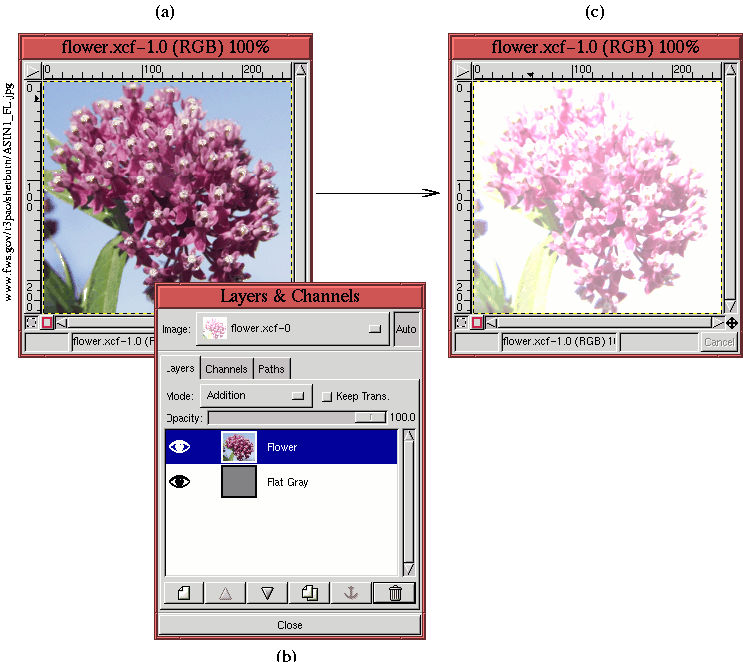illustrates an application of the Addition blending mode. Figure  5.15(a) displays the image of a flower, and Figure  5.15(b), showing the associated Layers dialog, indicates that this image consists of two-layers. The upper layer is the flower image, and the lower layer is filled with a medium gray whose pixel values are uniformly 127R 127G 127B.

Figure  5.15(a) shows the flower image for the Normal blending mode. Figure  5.15(c) shows the same image when the blending mode of the upper layer is changed to Addition. This has the effect of adding 127R 127G 127B to every pixel in the flower layer, which lightens the entire image considerably. In fact, some parts of the image are completely blown out to white.

The effect of the Subtract blending mode is illustrated in Figure  5.14(b). This blending mode works as follows. Given a foreground and background pixel, we'll again represent each as an RGB vector F=[r1,g1,b1] and B=[r2,g2,b2]. The pixel obtained from the Subtract blending mode is R=B-F=[r2-r1,g2-g1,b2-b1]. Thus, for the two blue arrows labeled F and B in Figure  5.14(b), the result of subtracting the foreground from the background is given by the red arrow labeled R.

Unlike the Addition blending mode, Subtract is not symmetrical (that is, subtracting F from B is not the same as subtracting B from F). The result of subtracting the foreground from the background can produce negative values. If a component of the resulting RGB vector is less than zero, it is clipped to the surface of the cube. Thus, the equation representing the Subtract blending mode is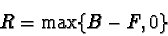where 0 represents the color 0R 0G 0B and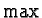is the function that performs component-wise maximization of the two vectors. Because the foreground color is always a positive number, the result is always darker than the background (unless either the foreground or background are black, and then there is no change).

The Difference blending mode is like Subtract, but the result is symmetrical between the foreground and the background. Difference is symmetrical because it applies an absolute value to the difference of the foreground and background values. Thus, if one of the RGB components is negative after subtraction, its sign is reversed to make it positive. The resulting mathematical expression for the Difference blending mode is

R=|F-B|

where the vertical bars in the equation represent the absolute value function.

Figure  5.16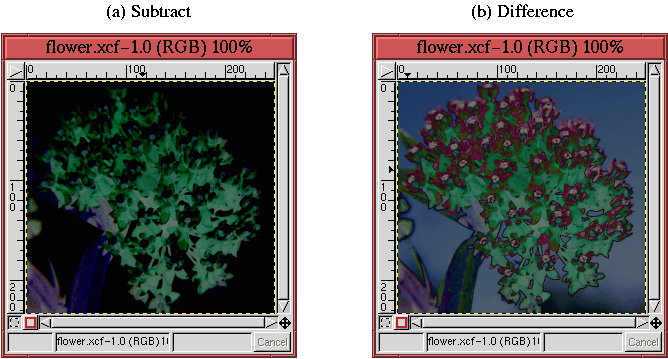illustrates the application of the Subtract and Difference blending modes. The modes are applied to the image from Figure  5.15(a), which consists of the flower in the upper layer and a medium gray in the lower layer. You can see that the result of using Subtract, shown in Figure  5.16(a), has regions that are totally black. This is where the difference between the foreground and background creates negative values that are clipped to zero. Difference, shown in Figure  5.16(b), however, has no clipped values because it employs the absolute value of the difference. Note that for both the Subtract and Difference modes, the results are darker than the original flower image.

Grokking The Gimp
Previous Page Home Next PagePublished under the terms of the Open Publication License Design by Interspire